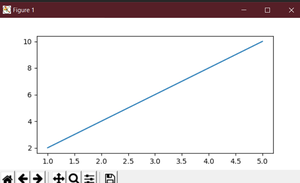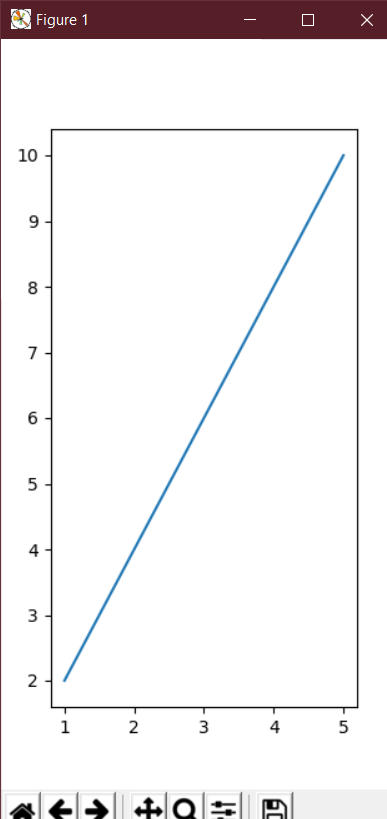Related Articles

# How to change the size of figures drawn with matplotlib?

• Difficulty Level : Medium
• Last Updated : 26 May, 2021

The main purpose of matplotlib is to create a figure representing data. The use of visualizing data is to tell stories by curating data into a form easier to understand, highlighting the trends and outliers. We can populate the figure with all different types of data, including axes, a graph plot, a geometric shape, etc. “When” we plot graphs we may want to set the size of a figure to a certain size. You may want to make the figure wider in size, taller in height, etc.

This can be achieved by an attribute of matplotlib known as figsize. The figsize attribute allows us to specify the width and height of a figure in unit inches.

Syntax:

import matplotlib.pyplot as plt

figure_name = plt.figure(figsize=(width, height))

The figsize attribute is a parameter of the function figure(). It is an optional attribute, by default the figure has the dimensions as (6.4, 4.8). This is a standard plot where the attribute is not mentioned in the function.

Normally each unit inch is of 80 x 80 pixels. The number of pixels per unit inch can be changed by the parameter dpi, which can also be specified in the same function.

Approach:

• We create a variable plt_1, and set it equal to, plt.figure(figsize=(6,3)).
• This creates a figure object, which has a width of 6 inches and 3 inches in height.
• The values of the figsize attribute are a tuple of 2 values.

Example 1:

## Python3

 `# We start by importing matplotlib``import` `matplotlib.pyplot as plt` `# Plotting a figure of width 6 and height 3``plt_1 ``=` `plt.figure(figsize``=``(``6``, ``3``))` `# Let's plot the equation y=2*x``x ``=` `[``1``, ``2``, ``3``, ``4``, ``5``]` `# y = [2,4,6,8,10]``y ``=` `[x``*``2` `for` `x ``in` `x]` `# plt.plot() specifies the arguments for x-axis``# and y-axis to be plotted``plt.plot(x, y)` `# To show this figure object, we use the line,``# fig.show()``plt.show()`

Output:

This works if you’re using a python IDE other than jupyter notebooks. If you are using

jupyter notebooks, then you would not use, plt.show(). Instead, you would specify in the

Code right after importing matplotlib, %matplotlib inline.Example 2:

To see the dynamic nature of figure sizing in matplotlib, now we to create a figure with the dimensions inverted. The height will now be double the size of the width.

## Python3

 `# We start by importing matplotlib``import` `matplotlib.pyplot as plt` `# Plotting a figure of width 3 and height 6``plt_1 ``=` `plt.figure(figsize``=``(``3``, ``6``))` `# Let's plot the equation y=2*x``x ``=` `[``1``, ``2``, ``3``, ``4``, ``5``]` `# y = [2,4,6,8,10]``y ``=` `[x``*``2` `for` `x ``in` `x]` `# plt.plot() specifies the arguments for``# x-axis and y-axis to be plotted``plt.plot(x, y)` `# To show this figure object, we use the line,``# fig.show()``plt.show()`

Output:Attention geek! Strengthen your foundations with the Python Programming Foundation Course and learn the basics.

To begin with, your interview preparations Enhance your Data Structures concepts with the Python DS Course. And to begin with your Machine Learning Journey, join the Machine Learning – Basic Level Course

My Personal Notes arrow_drop_up